# Equation

Equation
$-2{x}^{2}+bx-82=0$
has one root x1 = -8. Determine the coefficient b and the second root x2.

b =  -26.25
x2 =  -5.13

### Step-by-step explanation:Did you find an error or inaccuracy? Feel free to write us. Thank you!Tips to related online calculators

## Related math problems and questions:

• Roots and coefficient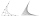In the equation 2x ^ 2 + bx-9 = 0 is one root x1 = -3/2. Determine the second root and the coefficient b.Quadratic equation 7x2+bx+c=0 has roots x1 = 67 and x2 = -84. Calculate the coefficients b and c.Determine the numbers b, c that the numbers x1 = -1 and x2 = 3 were roots of quadratic equation: -3x 2 + b x + c = 0
• Equation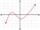Eequation f(x) = 0 has roots x1 = 64, x2 = 100, x3 = 25, x4 = 49. How many roots have equation f(x2) = 0 ?
• EQ2Solve quadratic equation: 2x²- 2(x-7)²+5x=0
• RootsDetermine the quadratic equation absolute coefficient q, that the equation has a real double root and the root x calculate: 5x 2 +9x + q = 0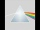Please determine the solvability conditions of the equation, solve the equation and perform the test: x divided by x squared minus 2x plus1 the whole minus x + 3 divided by x squared minus 1 this is equal to 0: x/(x2-2x+1) - (x+3)/( x2-1) = 0
• Parabola 3Find the equation of a parabola with its focus at (0,2) and its vertex at the origin. f: y=x2+bx+cSolve quadratic equation: 2x2+28x-550=0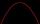Solve pure quadratic equation -5x2 +10 = 0.
• Eq2 equationsFor each of the following problems, determine the roots of the equation. Given the roots, sketch the graph and explain how your sketch matches the roots given and the form of the equation: g(x)=36x2-12x+5 h(x)=x2-4x+20 f(x)=4x2-24x+45 p(x)=9x2-36x+40
• CoefficientDetermine the coefficient of this sequence: 7.2; 2.4; 0.8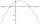It is given a quadratic function y = -4x2+5x+c with unknown coefficient c. Determine the smallest integer c for which the graph of f intersects the x-axis at two different points.Function f(x)=a(x-r)(x-s) the graph of the function has x- intercept at (-4, 0) and (2, 0) and passes through the point (-2,-8). Find constant a, r, s.If the square root of 3m2 +22 and -x = 0, and x=7, what is m?Solve the following equation with variations, combinations and permutations: 4 V(2,x)-3 C(2,x+ 1) - x P(2) = 0Calculate standard deviation for file: 63,65,68,69,69,72,75,76,77,79,79,80,82,83,84,88,90